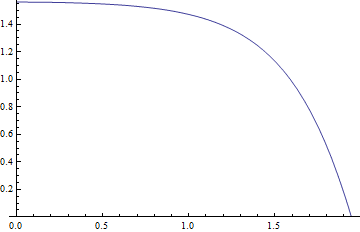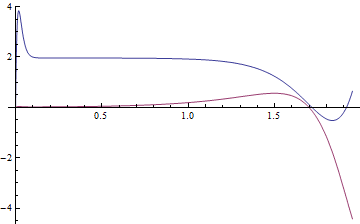# Free fall of hatch with a frictional hinge

Diego Saenz
Hi people!
I have a real life problem that i can't solve....Im trying to figure out the tangencial speed or angular velocity of a hatch at the moment it is about to close (initially it was closed). The hatch has been accelerating due to gravity. It looks much as a pendulum to me. But, it gets more complicated when you add the fact that the hinge of this hatch, has friction that can't be ignored.

- Can i calculate this friction?

- Can anybody help me to get a mathamatical model of this system? (maybe just doing energy balance).

- And the main question...How can i calculate the angular velocity at the moment of the impact with the frame?

Imagine something like this (http://www.getprice.com.au/images/uploadimg/592/_1_skyspan_metal_hatch.jpg [Broken])

Thanks.

Last edited by a moderator:

Angular velocity at impact is the easiest thing to do. Friction in the hinge provides constant (or close enough to) torque. So friction torque times angle from opened to closed gives you energy loss to friction. Compute difference in potential energies of hatch opened and closed, subtract off loss to friction, divide that by half the moment of inertia about hinge, and that will give you square of angular velocity.

You can do this to compute velocity as a function of angle through the whole swing. If you want angular velocity as function of time, or to get amount of time it takes the hatch to close, you'll have to solve a non-linear differential equation. There might be a solution for this, because there is a general solution for pendulum, but it's highly non-trivial. Your best bet is to solve it numerically.

VortexLattice
Depends how much you want to idealize it. If you say there's a constant friction and it's basically just a rectangle (and thus, just a rod really) around one axis, you can do it analytically very easily.

Homework Helper
Gold Member
I'm not so sure the hinge friction can be taken as constant. If the hinge is very tight that may be true, but if there's any slack it will fall off considerably as the hatch moves from vertical to horizontal. This is because the normal load will reduce.

VortexLattice
I'm not so sure the hinge friction can be taken as constant. If the hinge is very tight that may be true, but if there's any slack it will fall off considerably as the hatch moves from vertical to horizontal. This is because the normal load will reduce.

Definitely true. I was just giving an example. You could probably choose some very simple friction vs. angle relation and possibly get an analytic answer, still.

Homework Helper
Gold Member
You could probably choose some very simple friction vs. angle relation and possibly get an analytic answer, still.
A full analysis including friction looks nasty. If we ignore friction at first, we can compute the normal force at the hinge. When at angle θ to the vertical, I make it mg√(1-(15/16)sin2θ). The frictional loss of energy can then be obtained by multiplying by a constant and integrating for θ = 0 to π/2. So that should be valid for relatively low friction. OTOH, I haven't yet figured out how to integrate that analytically.

Normal force isn't going to be just a function of the angle. In fact, just before the hatch shuts, the force will change sign. If friction depends on normal force, this problem isn't going to be even close to solvable analytically. But that's what numerical diff. eq. solvers are for.

Homework Helper
Gold Member
Normal force isn't going to be just a function of the angle.
If θ is the angle to the vertical, 2r is the hatch width, H is the component of the normal force from the hinge along the line of the hatch and T is the component at right angles to the hatch:
H = mg cos(θ)
$mr\ddot{θ} = mg sin(θ) - T$
$I\ddot{θ} = mgr sin(θ)$
where I = (4/3)mr2
So $(4/3)mr\ddot{θ} = mg sin(θ)$
$T = mg sin(θ) - (3/4) mg sin(θ) = mg sin(θ)/4$
The normal force is therefore $\sqrt{H^2+T^2} = mg\sqrt{cos^2(θ)+sin^2(θ)/16}$
Is there a flaw in that?

voko
What about the air resistance? It seems it should no less important than the hinge friction.

Is there a flaw in that?
Yes. You are not working with constraint forces properly. The constraint is doing more than supporting the weight of the hatch. As the result the constraint at the hinge will depend not only on angle the hatch makes, but also on velocity and acceleration.

Here is a numerical solution for a frictionless hatch with finite angular momentum. θ(t)Fx(t) (red) and Fy(t) (blue)As you can see, the constraint switches from pushing to pulling right before the hatch slams shut. (The initial spike in Fy is due to settling in simulation.)